# McGraw Hill Math Grade 4 Chapter 5 Lesson 3 Answer Key Multiplying a Four-Digit Number

Practice the questions of McGraw Hill Math Grade 4 Answer Key PDF Chapter 5 Lesson 3 Multiplying a Four-Digit Number to secure good marks & knowledge in the exams.

## McGraw-Hill Math Grade 4 Answer Key Chapter 5 Lesson 3 Multiplying a Four-Digit Number

Multiply

Find each product.

Question 1.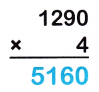1290 x 4 = 5160
Explanation:
4 × 0 = 0
4 × 9 = 36
Put the 6 in the appropriate column and carry the 3.
4 × 2 + 3(carried) = 11
Put the 1 in the appropriate column and carry the 1.
4 × 1 + 1(carried) = 5

Question 2.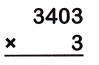Explanation:
3 × 3 = 9
3 × 0 = 0
3 × 4 = 12
Put the 2 in the appropriate column and carry the 1.
3 × 3 + 1(carried) = 10
Put the 0 in the appropriate column and carry the 1.

Question 3.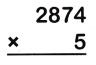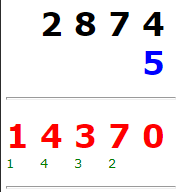Explanation:
5 × 4 = 20
Put the 0 in the appropriate column and carry the 2.
5 × 7 + 2(carried) = 37
Put the 7 in the appropriate column and carry the 3.
5 × 8 + 3(carried) = 43
Put the 3 in the appropriate column and carry the 4.
5 × 2 + 4(carried) = 14
Put the 4 in the appropriate column and carry the 1.

Question 4.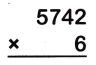Explanation:
6 × 2 = 12
Put the 2 in the appropriate column and carry the 1.
6 × 4 + 1(carried) = 25
Put the 5 in the appropriate column and carry the 2.
6 × 7 + 2(carried) = 44
Put the 4 in the appropriate column and carry the 4.
6 × 5 + 4(carried) = 34
Put the 4 in the appropriate column and carry the 3.

Question 5.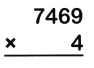Explanation:
4 × 9 = 36
Put the 6 in the appropriate column and carry the 3.
4 × 6 + 3(carried) = 27
Put the 7 in the appropriate column and carry the 2.
4 × 4 + 2(carried) = 18
Put the 8 in the appropriate column and carry the 1.
4 × 7 + 1(carried) = 29
Put the 9 in the appropriate column and carry the 2.

Question 6.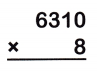Explanation:
8 × 0 = 0
8 × 1 = 8
8 × 3 = 24
Put the 4 in the appropriate column and carry the 2.
8 × 6 + 2(carried) = 50
Put the 0 in the appropriate column and carry the 5.

Question 7.
2,367 people visit a museum in the first week it opens. The same number come the second week. During the third week, 1,983 people visit. Circle the expression that shows how to find the total number of people who visited the museum in the first three weeks.
(2 × 2367) + 1983 2 × (2367 + 1983)
(2 × 1983) + 2367 2 × (1983 + 2367)